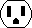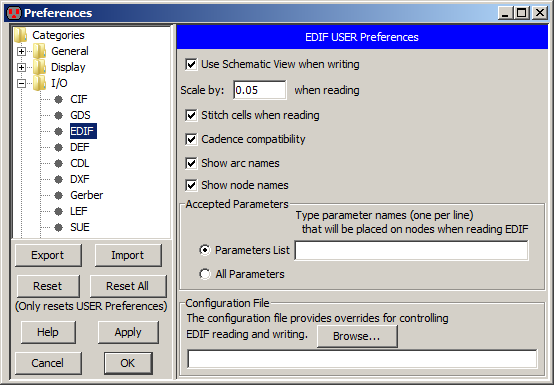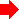Chapter 7: Technologies7-3: I/O Control 7-3-4: EDIF ControlFor more information on reading and writing EDIF, see Section 3-9-2 and Section 3-9-3, respectively. EDIF options are controlled with the EDIF Preferences (in menu File / Preferences..., "I/O" section, "EDIF" tab).These controls are supported by the dialog:

• Use Schematic View when writing controls whether EDIF output writes schematic or netlist views (the default is netlist).
• Scale by lets you set a scale factor for EDIF input.
• Stitch cells when reading invokes the Auto Stitching router after EDIF import to make explicit connections (see Section 9-6-2 for more)
• Cadence compatibility affects both EDIF input and output. When checked, output of multidimensional and symbolic busses is converted to simpler, all-numeric busses, and input of properties starting with "def" are added to cells as parameters.
• Show arc names and Show node names controls whether EDIF input makes arc and node names visible.
• Accepted Parameters lets you list those EDIF parameters that will be read (all others are ignored).

The bottom section of the panel lets you specify a configuration file that will control EDIF I/O. This file has conversions between coordinates and names inside of Electric and the EDIF file. The file has these lines of text that control different aspects of conversion:

• Primitives A line starting with "P" controls how primitives are converted to EDIF. The line has this format:
` P ElTech ElPrim ElFunc ElRot ElPortOff EdTech EdPrim EdFunc EdPortOff`
Where:
• `ElTech` is the Electric technology name (e.g. "schematic").
• `ElPrim` is the Electric primitive name (e.g. "Transistor").
• `ElFunc` is the Electric function (e.g. "CONPOWER").
• `ElRot` is the Electric rotation (e.g. "90").
• `ElPortOff` is the Electric port offsets, enclosed in braces (e.g. "{ g(-1,0) }"). The offsets are the values required to move the port to the origin, so if a port is at (2, -5), the offset should be (-2, 5). Each port on the primitive must be listed, and an offset given. To ignore a port, use "NA" instead of "port(x,y)". You can also specify an ignored port as "NA(x,y)" if you want to affect how an attached wire's endpoint is modified. "NA" by itself is the same as "NA(0,0)". If the port's name is "NA", use "\NA(x,y)" to specify the name as NA, and not be ignored.
• `EdTech` is the EDIF technology name (e.g. "tsmc18").
• `EdPrim` is the EDIF primitive name (e.g. "pmos2v").
• `EdFunc` is the EDIF function (e.g. "symbol").
• `EdPortOff` is the EDIF port offsets, enclosed in braces (e.g. "{ G(0,0) }"). Each port on the primitive must be listed, and an offset given. The offsets are the values required to move the port to the origin, so if a port is at (2, -5), the offset should be (-2, 5).
For example:
```P schematic Ground CONGROUND 0 { gnd(0,2) } basic gnd symbol { gnd!(0,0) } ```
• Cells A line starting with "C" controls how cells are converted to EDIF. The line has this format:
` C ElLib ElCell ElView ElRot ElPortOff EdTech EdPrim EdFunc EdPortOff`
Where:
• `ElLib` is the Electric library name (e.g. "MyCells").
• `ElCell` is the cell name in that library (e.g. "Inverter").
• `ElView` is the view name of the cell (e.g. "ic" for Icon).
All other fields are the same as in the "Primitive" line.
• Exports A line starting with "E" controls how exports are converted to EDIF. The line has this format:
` E ElTech ElPrim ElFunc ElRot ElPortOff EdTech EdPrim EdFunc EdPortOff`
Where:
• `ElTech` is the Electric technology name (e.g. "schematic").
• `ElPrim` is the Electric primitive name (e.g. "Transistor").
• `ElFunc` is the Electric function (e.g. "CONNECT").
• `ElRot` is the Electric rotation (e.g. "90").
• `ElPortOff` is the Electric port offsets, enclosed in braces (e.g. "{ g(-1,0) }"). The offsets are the values required to move the export to the origin, so if an export is at (2, -5), the offset should be (-2, 5). Each port on the primitive must be listed, and an offset given.
• `EdTech` is the EDIF technology name (e.g. "tsmc18").
• `EdPrim` is the EDIF primitive name (e.g. "pmos2v").
• `EdFunc` is the EDIF function (e.g. "symbol").
• `EdPortOff` is the EDIF port offsets, enclosed in braces (e.g. "{ G(0,0) }"). The offsets are the values required to move the export to the origin, so if an export is at (2, -5), the offset should be (-2, 5). Each port on the primitive must be listed, and an offset given.
For example:
```E schematic Off-Page CONNECT 0 input { a(-2,0), y(2,0) } basic ipin symbol { NA, NA } ```
• Variables A line starting with "V" controls how variables are converted to EDIF. The line has this format:
``` V ElVarName EdVarName Scale [Append] ```
Where:
• `ElVarName` is the Electric variable name (e.g. "ATTR_M").
• `EdVarName` is the EDIF primitive name (e.g. "m").
• `Scale` is a scale from Electric to EDIF (e.g. "1").
• `Append` is an optional string to append to EDIF (e.g. "u").
For example:
```V ATTR_length l 0.9 u ```
• FigureGroups A line starting with "F" controls how figure groups are converted to EDIF. The line has this format:
` F ElName EdName`
Where:
• `ElName` is the Electric technology name (e.g. "ARTWORK").
• `EdName` is the EDIF figure group name (e.g. "DEVICE").
For example:
```F ARTWORK DEVICE ```
• Globals A line starting with "G" controls how global names are converted to EDIF. The line has this format:
` G ElName EdName`
Where:
• `ElName` is the Electric global name (e.g. "GND").
• `EdName` is the EDIF global name (e.g. "gnd!").
For example:
```G GND gnd! ```PreviousTable of Contents Next